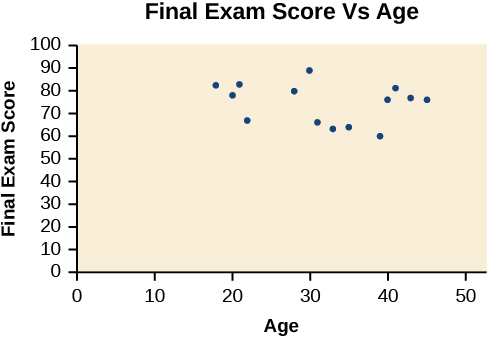# 4.3 Fitting linear models to data

 Page 1 / 14
In this section you will:
• Draw and interpret scatter diagrams.
• Use a graphing utility to find the line of best fit.
• Distinguish between linear and nonlinear relations.
• Fit a regression line to a set of data and use the linear model to make predictions.

A professor is attempting to identify trends among final exam scores. His class has a mixture of students, so he wonders if there is any relationship between age and final exam scores. One way for him to analyze the scores is by creating a diagram that relates the age of each student to the exam score received. In this section, we will examine one such diagram known as a scatter plot.

## Drawing and interpreting scatter plots

A scatter plot is a graph of plotted points that may show a relationship between two sets of data. If the relationship is from a linear model , or a model that is nearly linear, the professor can draw conclusions using his knowledge of linear functions. [link] shows a sample scatter plot.A scatter plot of age and final exam score variables

Notice this scatter plot does not indicate a linear relationship . The points do not appear to follow a trend. In other words, there does not appear to be a relationship between the age of the student and the score on the final exam.

## Using a scatter plot to investigate cricket chirps

[link] shows the number of cricket chirps in 15 seconds, for several different air temperatures, in degrees Fahrenheit Selected data from http://classic.globe.gov/fsl/scientistsblog/2007/10/. Retrieved Aug 3, 2010 . Plot this data, and determine whether the data appears to be linearly related.

 Chirps 44 35 20.4 33 31 35 18.5 37 26 Temperature 80.5 70.5 57 66 68 72 52 73.5 53

Plotting this data, as depicted in [link] suggests that there may be a trend. We can see from the trend in the data that the number of chirps increases as the temperature increases. The trend appears to be roughly linear, though certainly not perfectly so.

## Finding the line of best fit

Once we recognize a need for a linear function to model that data, the natural follow-up question is “what is that linear function?” One way to approximate our linear function is to sketch the line that seems to best fit the data. Then we can extend the line until we can verify the y -intercept. We can approximate the slope of the line by extending it until we can estimate the $\text{\hspace{0.17em}}\frac{\text{rise}}{\text{run}}.$

## Finding a line of best fit

Find a linear function that fits the data in [link] by “eyeballing” a line that seems to fit.

On a graph, we could try sketching a line. Using the starting and ending points of our hand drawn line, points (0, 30) and (50, 90), this graph has a slope of

$\begin{array}{l}\hfill \\ \begin{array}{l}m=\frac{60}{50}\hfill \\ \text{\hspace{0.17em}}\text{\hspace{0.17em}}\text{\hspace{0.17em}}\text{\hspace{0.17em}}=1.2\hfill \end{array}\hfill \end{array}$

and a y -intercept at 30. This gives an equation of

$T\left(c\right)=1.2c+30$

where $\text{\hspace{0.17em}}c\text{\hspace{0.17em}}$ is the number of chirps in 15 seconds, and $\text{\hspace{0.17em}}T\left(c\right)\text{\hspace{0.17em}}$ is the temperature in degrees Fahrenheit. The resulting equation is represented in [link] .

## Recognizing interpolation or extrapolation

While the data for most examples does not fall perfectly on the line, the equation is our best guess as to how the relationship will behave outside of the values for which we have data. We use a process known as interpolation when we predict a value inside the domain and range of the data. The process of extrapolation is used when we predict a value outside the domain and range of the data.

#### Questions & Answers

if sin15°=√p, express the following in terms of p
Norman Reply
prove sin²x+cos²x=3+cos4x
Kiddy Reply
the difference between two signed numbers is -8.if the minued is 5,what is the subtrahend
jeramie Reply
the difference between two signed numbers is -8.if the minuend is 5.what is the subtrahend
jeramie
what are odd numbers
micheal Reply
numbers that leave a remainder when divided by 2
Thorben
1,3,5,7,... 99,...867
Thorben
7%2=1, 679%2=1, 866245%2=1
Thorben
the third and the seventh terms of a G.P are 81 and 16, find the first and fifth terms.
Suleiman Reply
if a=3, b =4 and c=5 find the six trigonometric value sin
Martin Reply
ask
Ans
pls how do I factorize x⁴+x³-7x²-x+6=0
Gift Reply
in a function the input value is called
Rimsha Reply
how do I test for values on the number line
Modesta Reply
if a=4 b=4 then a+b=
Rimsha Reply
a+b+2ab
Kin
commulative principle
DIOSDADO
a+b= 4+4=8
Mimi
If a=4 and b=4 then we add the value of a and b i.e a+b=4+4=8.
Tariq
what are examples of natural number
sani Reply
an equation for the line that goes through the point (-1,12) and has a slope of 2,3
Katheryn Reply
3y=-9x+25
Ishaq
show that the set of natural numberdoes not from agroup with addition or multiplication butit forms aseni group with respect toaaddition as well as multiplication
Komal Reply
x^20+x^15+x^10+x^5/x^2+1
Urmila Reply
evaluate each algebraic expression. 2x+×_2 if ×=5
Sarch Reply

### Read also:

#### Get Jobilize Job Search Mobile App in your pocket Now!

Source:  OpenStax, Algebra and trigonometry. OpenStax CNX. Nov 14, 2016 Download for free at https://legacy.cnx.org/content/col11758/1.6
Google Play and the Google Play logo are trademarks of Google Inc.

Notification Switch

Would you like to follow the 'Algebra and trigonometry' conversation and receive update notifications?ByByBy OpenStaxBy OpenStaxBy OpenStaxBy Yasser IbrahimBy OpenStaxBy Angelica LitoBy Jazzycazz JacksonBy OpenStaxBy OpenStaxBy Brooke Delaney